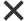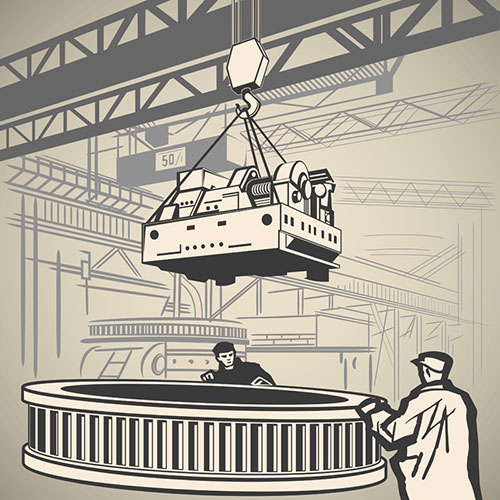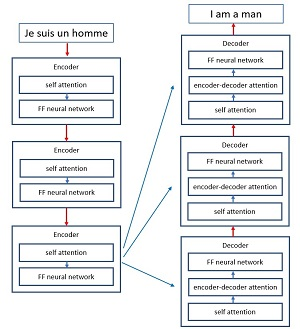### Neurosymbolic AI Advances State of the Art on Math Word ProblemsResearchers at Microsoft have demonstrated a new technique called Neurosymbolic AI which has shown promising results when applied to difficult scenarios such as algebra problems stated in words. The PureAI editors were given a sneak peek at the draft of a research paper that describes the work.

Machine learning and AI systems have made remarkable progress in many areas. Speech recognition from systems like Alexa, Siri, and Cortana are now routinely taken for granted. Image classification and facial recognition systems are coming close to human performance. Specific types of natural language processing, such as sentiment analysis systems that classify if a written movie review is positive, negative, or neutral are often impressively accurate.

However, some problem scenarios remain extremely challenging. Many of these difficult scenarios have a combination of natural language and reasoning. For example, consider this problem, "Suppose 0 = 2*a + 3*a − 150. Let p = 106 − 101. Suppose −3*b + w + 544 = 3*w, −p*b − 5*w = −910. What is the greatest common factor of b and a?" In case your algebra is a bit rusty, the correct answer is 30. A fairly difficult problem.

And consider this question, "Let r(g) be the second derivative of 2*g**3/3 − 21*g**2/2 + 10*g. Let z be r(7). Factor −z*s + 6 − 9*s**2 + 0*s + 6*s**2." The answer to this problem is −(s + 3)*(3*s − 2). A difficult problem for both man and machine.

Some recent work at Microsoft Research has demonstrated significant progress at tackling such problems using a new approach called Neurosymbolic AI. The research article will eventually be published on the Microsoft Research blog site.

The math problems above are part of a set of benchmark problems called, somewhat uninspiringly, the Mathematics Dataset. The dataset contains 16 million question-answer pairs in the following eight categories:

1. algebra (linear equations, polynomial roots, sequences)
2. arithmetic (pairwise operations and mixed expressions, square roots, cube roots)
3. calculus (differentiation)
4. comparison (closest numbers, pairwise comparisons, sorting)
5. measurement (conversion, working with time)
6. numbers (base conversion, remainders, common divisors and multiples, prime numbers, place value, rounding)
7. polynomials (addition, simplification, composition, evaluating, expansion)
8. probability (sampling without replacement)

The current state of the art for the benchmark dataset is 76 percent accuracy. For an answer to be marked as correct, it must match the answer in the dataset perfectly, character for character. The Neurosymbolic AI approach significantly improved upon the 76 percent accuracy mark (you can read about the exact improvement when the article is published).

The Microsoft researchers also applied the Neurosymbolic AI approach to a second benchmark set, the MathQA Dataset. The MathQA Dataset is a formatting wrapper around the AQuA-RAT (Algebra Question Answering with Rationales) Dataset. An example problem is:

```{
"question": "A grocery sells a bag of ice for \$1.25, and makes 20 percent profit.
If it sells 500 bags of ice, how much total profit does it make?",

"options": ["A)125", "B)150", "C)225", "D)250", "E)275"],

"rationale": "Profit per bag = 1.25 * 0.20 = 0.25\nTotal profit = 500 * 0.25

"correct": "A"
}```

There are about 100,000 problems in the dataset. Instead of just coming up with an answer, the goal is to produce a sequence of steps that solves the problem. In order for an answer to be marked as correct, it must match the answer in the database exactly.

Current state of the art for the MathQA / AQuA-RAT dataset is 59 percent accuracy. The Neurosymbolic AI approach significantly improved upon this accuracy figure.

Dr. Paul Smolensky, the Microsoft lead researcher of the effort, commented, "I do believe that Neurosymbolic AI may be a significant addition to our understanding of these types of problems."

The new Neurosymbolic AI approach used by Microsoft Research essentially combines two existing techniques: neural attention Transformers (the "Neuro" part of Neurosymbolic AI) and tensor product representation (the "-symbolic" part).

Understanding Neural Transformers
Solving a math word problem is a type of machine learning sequence-to-sequence task. The input is a sequence of symbols (letters, digits, and punctuation) and the output is a sequence of symbols. Another type of sequence-to-sequence problem is language translation, for example, translating "Je suis un homme" to "I am a man."

Over the past few years, several different neural network architectures have been applied to sequence-to-sequence problems. These techniques include standard deep neural networks, simple recurrent neural networks, LSTM (long short-term memory) neural networks, and convolutional neural networks (CNNs).

While all of these neural architectures give reasonably good results when applied to relatively simple sequence-to-sequence problems, they typically fail when presented with difficult tasks such as math word problems. In essence the architectures process one word at a time in a sequential way: "Je" -> "suis" -> "un" -> "homme" where each word is encoded as a numeric vector of roughly 100 to 500 values called a word embedding: "Je" = (0.3427, 1.0936, . . ).

A relatively new neural architecture called a neural Transformer combines elements of several neural network architectures. The two key concepts of Transformer architectures are that they add an attention component by looking at all the words in sentence as a whole rather than just looking at a stream of individual words, and the architectures are designed to allow parallel processing to speed up model training. A high-level block diagram of a Transformer architecture is shown in Figure 1.[Click on image for larger view.] Figure 1: Attention Transformer Neural Architecture

Dr. James McCaffrey, a Microsoft research engineer, commented, "Neural attention transformer architectures are some of the most fascinating and complex software components I've seen."

A Transformer model is a sequence of conceptual layers, where each layer can be thought of as a memory component. In each memory layer, there is a memory-object that corresponds to each word in the input sentence. Each memory-object contains a retrieval key and an associated value.

At each layer, each memory-object issues a set of queries to the memory bank for its layer, and for each query, the memory returns a result which is a weighted sum of all its values, according to how well the key matches the query. This is a value that indicates how much attention should be paid to each word in the input sentence.

Understanding Tensor Product Representations
A tensor product representation (TPR) is a way to express a relationship between symbols. The scheme is very clever and is best explained by example.

Suppose you have n = 4 items and you want to express a binary relationship between pairs of the items. Let the 4 items be expressed as tensors (vectors) with 4 values each:

```goats = ( 1,  1,  1,  1 )
grass = ( 1,  1, -1, -1 )
voles = ( 1, -1, -1,  1 )
roots = ( 1, -1,  1, -1 )
```

Each tensor is specially constructed so that they're orthonormal to each other, constructed using Hadamard matrices. And now suppose you have two "eat" relationships, (goats x grass) and (voles x roots). The relationships are symmetric so "goats eat grass" and "grass is eaten by goats" are two ways to interpret the relationship.

To express (goats x grass) you compute the matrix product of goats and grass (or grass and goats):

```  1   *  ( 1  1  1  1 )    1  1  1  1
1                        1  1  1  1
-1                       -1 -1 -1 -1
-1                       -1 -1 -1 -1

(grass)   (goats)
4x1       1x4              4x4```

Note: For simplicity some constants are being left out. For example, in order to normalize each row vector, you divide by square root of the dimension. So the vector tor for grass is really (0.5, 0.5, -0.5, -0.5), or k(1, 1, -1, -1) where k = 1/2.

And to express (voles x roots) you compute the matrix product of voles and roots (or roots and voles):

```  1   *  ( 1 -1 -1  1 )    1 -1 -1  1
-1                       -1  1  1 -1
1                        1 -1 -1  1
-1                       -1  1  1 -1

(roots)   (voles)
4x1       1x4              4x4```

In short, each relationship is represented by a matrix product of tensors -- hence the name tensor product representation.

Now the interesting thing is that you can answer questions like, "What do goats eat?"

```  1  1  1  1    *  ( 1 1 1 1 )T  = ( 1  1 -1 -1 )T
1  1  1  1
-1 -1 -1 -1
-1 -1 -1 -1

(goats x grass)     (goats)      =  (grass)
4x4                 4x1             4x1```

Here the upper case "T" indicates matrix transpose. Furthermore, you can represent (goats x grass AND voles x roots) by matrix addition of the two individual relationships and still answer "What do goats eat?" Quite remarkable.

There are many additional details about TPRs, for example, you can have multi-dimensional relationships, such as a three-dimensional relationship like person A bought item X at location Z.

Wrapping Up
Separately, attention Transformer neural architectures and system based on tenor product representations have been somewhat successful at solving difficult sequence-to-sequence problems such as math word problems and language translation. But when the two techniques are combined, early research evidence suggests that the hybrid architecture gives a significant improvement in accuracy.

Dr. McCaffrey commented, "The Neurosymbolic AI scheme of combining complex connectionist neural architectures with tensor product representation symbolic information is elegant and fascinating. Dr. Smolensky and his colleagues have created an intriguing new approach for sequence-to-sequence reasoning and I'm looking forward to reading their full research paper when it becomes available."